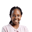Related Tags

scipy
communitycreator

# How to use the scipy.interpolate.griddata() methodJoy Kareko

### Overview

Scipy is a Python library useful for scientific computing. It contains numerous modules, including the interpolate module, which is helpful when it comes to interpolating data points in different dimensions whether one-dimension as in a line or two-dimension as in a grid.

The scipy.interpolate.griddata() method is used to interpolate on a 2-Dimension grid.

### Syntax

The syntax is as below:

scipy.interpolate.griddata(points, values, xi, method='linear',
fill_value=nan, rescale=False)



### Parameters

• points means the randomly generated data points.

• values are data points generated using a function.

• xi are the grid data points to be used when interpolating.

• method means the method of interpolation. It can be cubic, linear or nearest.

• The fill_value, which defaults to nan if the specified points are out of range. However, for nearest, it has no effect.

• rescale is useful when some points generated might be extremely large. In that case, it is set to True.

### Return value

The function returns an array of interpolated values in a grid.

### Code

import numpy as np
import matplotlib.pyplot as plt
import scipy
from scipy.interpolate import griddata

#define a function
def func(x,y):
return (x**2+y**2+(x*y)**2)**2

#generate grid data using mgrid
grid_x,grid_y = np.mgrid[0:1:1000j, 0:1:2000j]

#generate random points
rng = np.random.default_rng()
points = rng.random((1000, 2))

#generate values from the points generated above
values = func(points[:,0], points[:,1])

#generate grid data using the points and values above
grid_a = griddata(points, values, (grid_x, grid_y), method='cubic')

grid_b = griddata(points, values, (grid_x, grid_y), method='linear')

grid_c = griddata(points, values, (grid_x, grid_y), method='nearest')

#visualizations
fig, axs = plt.subplots(2, 2)
axs[0, 0].plot(func(grid_x,grid_y))
axs[0, 0].set_title("main")
axs[1, 0].plot(grid_a)
axs[1, 0].set_title("cubic")
axs[0, 1].plot(grid_b)
axs[0, 1].set_title("linear")
axs[1, 1].plot(grid_c)
axs[1, 1].set_title("nearest")
fig.tight_layout()

plt.savefig('output/graph.png')
Using the scipy.interpolate.griddata() method

### Explanation

Here is a line-by-line explanation of the code above:

• Lines 1–4: We import the necessary modules.
• Lines 8 and 9: We define a function that will be used to generate values later in line 19.
• Line 12: We generate grid data and return a 2-D grid.
• Line 15: We initialize a generator object for generating random numbers.
• Line 16: We use the generator object in line 15 to generate 1000, 2-D arrays.
• Line 20: We generate values using the points in line 16 and the function defined in lines 8-9.
• Lines 23–27: We generate grid points using the griddata method, over different grids, specifying different method parameters.
• Lines 31–42: We plot the different griddata visualizations.

RELATED TAGS

scipy
communitycreator

CONTRIBUTORJoy Kareko
RELATED COURSES

View all Courses

Keep Exploring

Learn in-demand tech skills in half the time